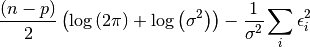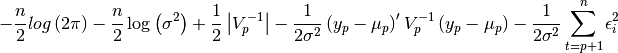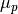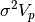# statsmodels.tsa.ar_model.AR.loglike¶

AR.loglike(params)[source]

The loglikelihood of an AR(p) process

Parameters: params : array The fitted parameters of the AR model llf : float The loglikelihood evaluated at params

Notes

Contains constant term. If the model is fit by OLS then this returns the conditonal maximum likelihood.If it is fit by MLE then the (exact) unconditional maximum likelihood is returned.whereis a (p x 1) vector with each element equal to the mean of the AR process andis the (p x p) variance-covariance matrix of the first p observations.